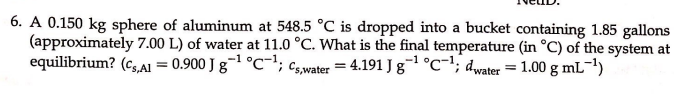# 6. A 0.150 kg sphere of aluminum at 548.5 degrees C is dropped into a bucket containing 1.85 gallons (approximately 7.00 L) of water at 11.0 degrees C. What is the final temperature (i degrees C) of the system at equilibrium? (Cs,Al = 0.900 J g^-1 degrees C^-1, Cs,water = 4.191 J g^-1 degrees C6-1; dwater = 1.00 g mL^-1)